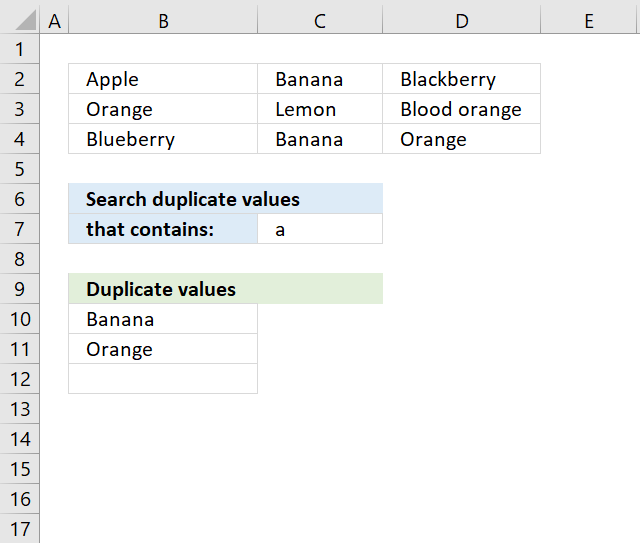Author: Oscar Cronquist Article last updated on December 11, 2018The array formula in cell B10 extracts duplicate values from cell range B2:D4 if they contain string specified in cell C7.

Array formula in B10:

=TEXTJOIN("", TRUE, IF(MIN(IF((COUNTIF(B9:\$B\$9, \$B\$2:\$D\$4)=0)*ISNUMBER(SEARCH(\$C\$7, \$B\$2:\$D\$4))*(COUNTIF(\$B\$2:\$D\$4, \$B\$2:\$D\$4)>1), (ROW(\$B\$2:\$D\$4)+(1/(COLUMN(\$B\$2:\$D\$4)+1)))*1, ""))=(ROW(\$B\$2:\$D\$4)+(1/(COLUMN(\$B\$2:\$D\$4)+1)))*1, \$B\$2:\$D\$4, ""))

To enter an array formula, type the formula in a cell then press and hold CTRL + SHIFT simultaneously, now press Enter once. Release all keys.

The formula bar now shows the formula with a beginning and ending curly bracket telling you that you entered the formula successfully. Don't enter the curly brackets yourself.

### Explaining formula in cell B10

#### Step 1 - Identify values containing search string

The SEARCH function returns a number representing the location of a text string in another string.

ISNUMBER(SEARCH(\$C\$7,\$B\$2:\$D\$4))

becomes

ISNUMBER(SEARCH("a",{"Apple", "Banana", "Blackberry"; "Orange", "Lemon", "Blood orange"; "Blueberry", "Banana", "Orange"}))

becomes

ISNUMBER({1,2,3;3,#VALUE!,9;#VALUE!,2,3})

and returns

{TRUE,TRUE, TRUE;TRUE, FALSE,TRUE; FALSE,TRUE, TRUE}

#### Step 2 - Keep track of previous values

The COUNTIF function counts values based on a condition or criteria, the first argument contains an expanding cell reference, it grows when the cell is copied to cells below. This makes the formula aware of values displayed in cells above. 0 (zero) indicates values that not yet have been displayed

COUNTIF(B9:\$B\$9,\$B\$2:\$D\$4)=0

becomes

COUNTIF("Unique distinct values", {"Apple", "Banana", "Blackberry";"Orange", "Lemon", "Blood orange";"Blueberry", "Banana", "Orange"})=0

becomes

{0,0,0;0,0,0;0,0,0}=0

and returns

{TRUE,TRUE,TRUE; TRUE,TRUE,TRUE; TRUE,TRUE,TRUE}.

#### Step 3 - Identify duplicates

The COUNTIF function counts values based on a condition or criteria, a value is larger than 1 indicates a dupilcate.

COUNTIF(\$B\$2:\$D\$4,\$B\$2:\$D\$4)>1

becomes

COUNTIF({"Apple", "Banana", "Blackberry";"Orange", "Lemon", "Blood orange";"Blueberry", "Banana", "Orange"}, {"Apple", "Banana", "Blackberry";"Orange", "Lemon", "Blood orange";"Blueberry", "Banana", "Orange"})>1

becomes

{1,2,1;2,1,1;1,2,2}>1

and returns

{FALSE,TRUE, FALSE;TRUE, FALSE,FALSE; FALSE,TRUE, TRUE}.

#### Step 4 - Multiply arrays

All three corresponding values must be true in order to get the value in a later step.

(COUNTIF(B9:\$B\$9, \$B\$2:\$D\$4)=0)*ISNUMBER(SEARCH(\$C\$7, \$B\$2:\$D\$4))*(COUNTIF(\$B\$2:\$D\$4, \$B\$2:\$D\$4)>1)

becomes

{TRUE,TRUE, TRUE;TRUE, FALSE,TRUE; FALSE,TRUE, TRUE}*{TRUE,TRUE,TRUE; TRUE,TRUE,TRUE; TRUE,TRUE,TRUE}* {FALSE,TRUE, FALSE;TRUE, FALSE,FALSE; FALSE,TRUE, TRUE}

and returns

{0,1,0; 1,0,0; 0,1,1}

#### Step 5 - Replace TRUE with unique number

The IF function returns a unique number if boolean value is TRUE. FALSE returns "" (nothing). The unique number is needed to find the right value in a later step.

IF((COUNTIF(B9:\$B\$9, \$B\$2:\$D\$4)=0)*ISNUMBER(SEARCH(\$C\$7, \$B\$2:\$D\$4))*(COUNTIF(\$B\$2:\$D\$4, \$B\$2:\$D\$4)>1), (ROW(\$B\$2:\$D\$4)+(1/(COLUMN(\$B\$2:\$D\$4)+1)))*1, "")

becomes

IF({0,1,0; 1,0,0; 0,1,1}, (ROW(\$B\$2:\$D\$4)+(1/(COLUMN(\$B\$2:\$D\$4)+1)))*1, "")

becomes

IF({0,1,0; 1,0,0; 0,1,1}, {2.33333333333333, 2.25, 2.2; 3.33333333333333, 3.25, 3.2; 4.33333333333333, 4.25, 4.2}, "")

and returns

{"",2.25,""; 3.33333333333333,"",""; "",4.25,4.2}

#### Step 6 - Find smallest value in array

The MIN function returns the smallest number in array ignoring blanks and text values.

MIN(IF((COUNTIF(B9:\$B\$9, \$B\$2:\$D\$4)=0)*ISNUMBER(SEARCH(\$C\$7, \$B\$2:\$D\$4))*(COUNTIF(\$B\$2:\$D\$4, \$B\$2:\$D\$4)>1), (ROW(\$B\$2:\$D\$4)+(1/(COLUMN(\$B\$2:\$D\$4)+1)))*1, ""))

becomes

MIN({"",2.25,""; 3.33333333333333,"",""; "",4.25,4.2})

and returns 2.25.

#### Step 7 - Find corresponding value

IF(MIN(IF((COUNTIF(B9:\$B\$9, \$B\$2:\$D\$4)=0)*ISNUMBER(SEARCH(\$C\$7, \$B\$2:\$D\$4))*(COUNTIF(\$B\$2:\$D\$4, \$B\$2:\$D\$4)>1), (ROW(\$B\$2:\$D\$4)+(1/(COLUMN(\$B\$2:\$D\$4)+1)))*1, ""))=(ROW(\$B\$2:\$D\$4)+(1/(COLUMN(\$B\$2:\$D\$4)+1)))*1,\$B\$2:\$D\$4,"")

becomes

IF(2.2={2.33333333333333, 2.25, 2.2; 3.33333333333333, 3.25, 3.2; 4.33333333333333, 4.25, 4.2}, \$B\$2:\$D\$4, "")

becomes

IF({FALSE,FALSE,TRUE; FALSE,FALSE,FALSE; FALSE,FALSE,FALSE}, \$B\$2:\$D\$4, "")

and returns

{"","","Banana";"","","";"","",""}

#### Step 8 - Concatenate strings in array

The TEXTJOIN function returns values concatenated ignoring blanks in array.

TEXTJOIN("",TRUE,IF(MIN(IF((COUNTIF(B9:\$B\$9,\$B\$2:\$D\$4)=0)*ISNUMBER(SEARCH(\$C\$7,\$B\$2:\$D\$4)),(ROW(\$B\$2:\$D\$4)+(1/(COLUMN(\$B\$2:\$D\$4)+1)))*1,""))=(ROW(\$B\$2:\$D\$4)+(1/(COLUMN(\$B\$2:\$D\$4)+1)))*1,\$B\$2:\$D\$4,""))

becomes

TEXTJOIN("", TRUE, {"","","Banana";"","","";"","",""})

and returns "Banana" in cell B10.